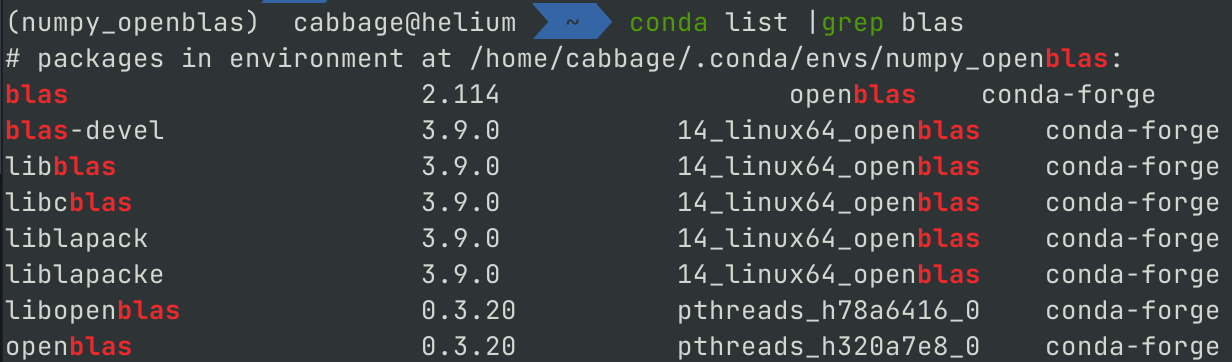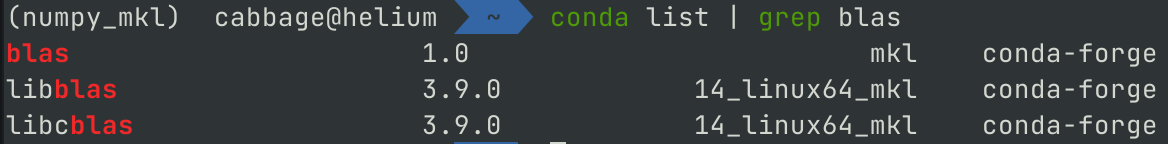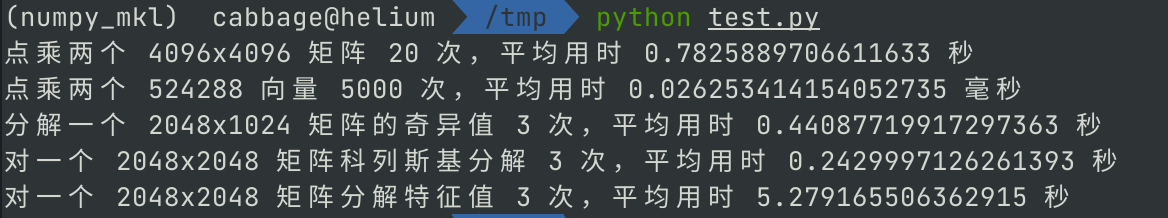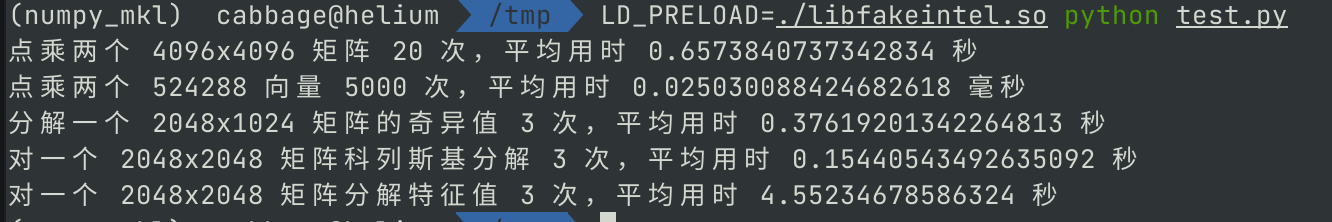Zen 系列 CPU 近五年多来可以说越来越香，游戏、直播、视频…… 不管什么平台什么用途，在 Intel 12 代以前基本都是首选 AMD。

### 1. 背景

Linux 上基本是这样操作的，先写个函数 `fakeintel.c`

``````int mkl_serv_intel_cpu_true() { return 1; }
``````

``````gcc -shared -fPIC -o libfakeintel.so fakeintel.c
``````

``````LD_PRELOAD=./libfakeintel.so python test.py
``````

### 2. 安装环境

Python 环境中要查看 blas 库信息，一般是用 `numpy` 带的那个打印 blas 信息的函数 `np.show_config`，不过实测可能并不准确。下面讲下如何正确安装链接了 mkl 的 numpy。

``````conda create python numpy "libblas=*=*openblas" "blas=*=*openblas" -n numpy_openblas
``````

``````conda activate numpy_openblas
conda list | grep blas
````````````blas_mkl_info:
libraries = ['blas', 'cblas', 'lapack', 'pthread', 'blas', 'cblas', 'lapack']
library_dirs = ['/home/cabbage/.conda/envs/numpy_openblas/lib']
define_macros = [('SCIPY_MKL_H', None), ('HAVE_CBLAS', None)]
include_dirs = ['/home/cabbage/.conda/envs/numpy_openblas/include']
blas_opt_info:
libraries = ['blas', 'cblas', 'lapack', 'pthread', 'blas', 'cblas', 'lapack', 'blas', 'cblas', 'lapack']
library_dirs = ['/home/cabbage/.conda/envs/numpy_openblas/lib']
define_macros = [('SCIPY_MKL_H', None), ('HAVE_CBLAS', None)]
include_dirs = ['/home/cabbage/.conda/envs/numpy_openblas/include']
lapack_mkl_info:
libraries = ['blas', 'cblas', 'lapack', 'pthread', 'blas', 'cblas', 'lapack']
library_dirs = ['/home/cabbage/.conda/envs/numpy_openblas/lib']
define_macros = [('SCIPY_MKL_H', None), ('HAVE_CBLAS', None)]
include_dirs = ['/home/cabbage/.conda/envs/numpy_openblas/include']
lapack_opt_info:
libraries = ['blas', 'cblas', 'lapack', 'pthread', 'blas', 'cblas', 'lapack', 'blas', 'cblas', 'lapack']
library_dirs = ['/home/cabbage/.conda/envs/numpy_openblas/lib']
define_macros = [('SCIPY_MKL_H', None), ('HAVE_CBLAS', None)]
include_dirs = ['/home/cabbage/.conda/envs/numpy_openblas/include']
``````

``````conda deactivate
conda create python numpy "libblas=*=*mkl" "blas=*=*mkl" -n numpy_mkl
conda activate numpy_mkl
``````### 3. 测试用的代码

``````import numpy as np
from time import time
#print('Numpy config:')
#np.show_config()

# 生成测试数据，控制变量，非随机
np.random.seed(0)
size = 4096
A, B = np.random.random((size, size)), np.random.random((size, size))
C, D = np.random.random((size * 128,)), np.random.random((size * 128,))
E = np.random.random((size // 2, size // 4))
F = G = np.random.random((size // 2, size // 2))
F = np.dot(F, F.T)

# 矩阵点乘
def dot(N=20):
t = time()
for i in range(N):
np.dot(A, B)
print(f'点乘两个 {size}x{size} 矩阵 {N} 次，平均用时 {(time() - t) / N} 秒')

# 向量乘法
def v_dot(N=5000):
t = time()
for i in range(N):
np.dot(C, D)
print(f'点乘两个 {size * 128} 向量 {N} 次，平均用时 {(time() - t) / N * 1000} 毫秒')

# 奇异值分解
def svd(N=3):
t = time()
for i in range(N):
np.linalg.svd(E, full_matrices=False)
print(f'分解一个 {size // 2}x{size // 4} 矩阵的奇异值 {N} 次，平均用时 {(time() - t) / N} 秒')

# 科列斯基分解
def cholesky(N=3):
t = time()
for i in range(N):
np.linalg.cholesky(F)
print(f'对一个 {size // 2}x{size // 2} 矩阵科列斯基分解 {N} 次，平均用时 {(time() - t) / N} 秒')

# 特征分解
def eig(N=3):
t = time()
for i in range(N):
np.linalg.eig(G)
print(f'对一个 {size // 2}x{size // 2} 矩阵分解特征值 {N} 次，平均用时 {(time() - t) / N} 秒')

if __name__ == '__main__':
dot()
v_dot()
svd()
cholesky()
eig()
``````

### 4. 运行各 blas 库的 numpy 测试

• 使用 OpenBLAS 的 numpy

``````conda activate numpy_openblas
python test.py
``````• mkl 的 numpy，不强制开启完整支持

``````conda activate numpy_mkl
python test.py
``````• mkl 的 numpy，用上边提到动态库劫持的方法强制开启完整支持

``````conda activate numpy_mkl